## QED

### QED Lagrangian

1. Full form of the QED Lagrangian
2. Mass of the Photon field
3. Intermezzo 1: P and C of the photon field
4. Intermezzo 2: Polarisation vectors of the photon field
Solutions of the wave equation for the electromagnetic four-potential in the Lorentz gauge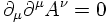are given by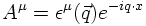where the four-vector εμ is the polarisation vector of the photon and only depends on the three-momentum of the photon since q2=0.

The polarisation vector describes a spin-1 boson but has four components!
How is this possible?

First, the Lorentz gauge results in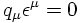reducing the number of independent components to three.

There is still the freedom to make an additional gauge transformation: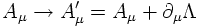with Λ being an arbitrary scalar function satisfying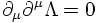so that the Lorentz condition is still valid.

Chosing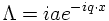the physics remains unchanged by the transformation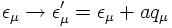This gives the freedom to set ε0=0 so that the Lorentz condition reads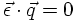This noncovariant gauge is called the Coulomb gauge.

As a consequence, there are only two independent photon polarisation vectors, both being transverse to the three-momentum vector of the photon.
For a photon travelling in the z direction one may choose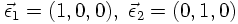The following linear combinations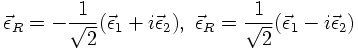describe photons of helicity +1 or -1, respectively.
This can be seen by considering a rotation around the z axis.
These polarisation vectors are also called circular polarisation vectors.